# Why & How to Use Window Functions to Aggregate Data in Postgres

Created on Dec 31, 2022

A window function is a feature developed in PostgreSQL — available since version 8.4 — to analyze data beyond the current row (hence the term “window”).

These windows can aggregate information to each row of your output. Apart from aggregate functions and groupings, window functions provide another way to perform calculations based on the values of several records.

Using window functions removes the hassle of using subqueries and `JOINS ` to aggregate neighboring rows that can be related to the current row.

This tutorial will show you how aggregate functions are used as window functions. You will learn how to use ` MAX() ` , ` MIN() ` , `AVG() ` , ` SUM() ` , ` ROW_NUMBER() ` , ` RANK() ` , and `DENSE_RANK() ` as window functions. But first, you need to know why you should use them.

✅ You can use the CoderPad sandbox in a new browser window to run the queries in this tutorial — the table is already loaded for you!

## Why use window functions

To get an idea of why you should use window functions, let’s start with an example database: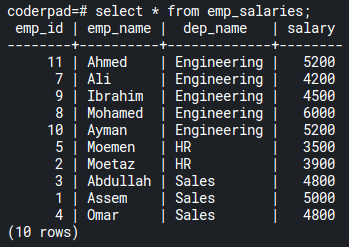Using this data, we want to get a breakdown of salaries for each employee in every department. We want to analyze the max salary across each department to compare it with each employee record.

To get that query result, you would probably use a Common Table Expression (CTE) with a ` JOIN ` statement like the following:

``````WITH dep_stats AS (
SELECT dep_name, max(salary) max_salary
FROM emp_salaries
GROUP BY dep_name
)

SELECT e.*, d.max_salary
FROM emp_salaries e
LEFT OUTER JOIN dep_stats d ON d.dep_name = e.dep_name;
``````

That query will give you this output: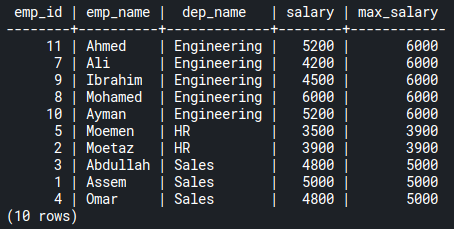But a better way to get the same result is to use a window function like the following:

``````SELECT e.*,
MAX(salary) OVER(PARTITION BY dep_name) max_salary
FROM emp_salaries e;
``````

As you can see, using a window function makes writing complex SQL queries easy. It allows you to output the current rows and add aggregate values accordingly.

ℹ️ Notice that window functions allowed us to perform aggregation without using the ` GROUP BY ` clause, as in the case of aggregate functions.

## Create a sample table

In this tutorial, you can experiment with your own table or create a sample table that we will borrow from the PostgreSQL documentation with a simple tweak. If you’re doing this tutorial from your own IDE, you can create your sample table by running the following script:

``````DROP TABLE IF EXISTS emp_salaries;

CREATE TABLE emp_salaries (
emp_id SERIAL PRIMARY KEY,
emp_name VARCHAR(30),
dep_name VARCHAR(40),
salary DECIMAL
);

INSERT INTO emp_salaries VALUES (11, 'Ahmed', 'Engineering', 5200);
INSERT INTO emp_salaries VALUES (7, 'Ali', 'Engineering', 4200);
INSERT INTO emp_salaries VALUES (9, 'Ibrahim', 'Engineering', 4500);
INSERT INTO emp_salaries VALUES (8, 'Mohamed', 'Engineering', 6000);
INSERT INTO emp_salaries VALUES (10, 'Ayman', 'Engineering', 5200);
INSERT INTO emp_salaries VALUES (5, 'Moemen', 'HR', 3500);
INSERT INTO emp_salaries VALUES (2, 'Moetaz', 'HR', 3900);
INSERT INTO emp_salaries VALUES (3, 'Abdullah', 'Sales', 4800);
INSERT INTO emp_salaries VALUES (1, 'Assem', 'Sales', 5000);
INSERT INTO emp_salaries VALUES (4, 'Omar', 'Sales', 4800);
``````

⚠️ If you’re testing in your own environment, you’ll drop a table of the ` emp_salaries ` name if it does exist.

Alternatively if you want to skip the table creation task, you can use the CoderPad sandbox to run the rest of the tutorial, as the sandbox already has the table loaded for you.

## PostgreSQL window function syntax

The syntax of a window function is simple; it looks something like this:

` <function_name>(<argument(s)>) OVER(PARTITION BY <column(s)> ORDER BY <column(s)>) <alias> `

The ` <function_name> ` is an aggregate function like ` SUM() ` , `COUNT() ` , ` MAX() ` , ` MIN() ` , ` AVG() ` , ` RANK() ` , etc. This function can take a column as an argument and can also take other arguments depending on the use case.

The ` OVER() ` clause defines the window size. If it’s empty and `PARTITION BY ` and ` ORDER BY ` are omitted, it means the window is across the whole table. However, ` PARTITION BY ` partitions the window by the column(s) that follow the clause. In addition, ` ORDER BY` sorts the partitioned window by the column(s) indicated by ` ORDER BY ` .

## PostgreSQL window functions examples

To get an idea of how to best use window functions, we’ll start with a simple SQL query using an aggregate function:

``````SELECT MAX(salary) max_salary
FROM emp_salaries;
``````

This query outputs the maximum salary across all the records.

What if you want this max value next to each record in the table?

You might say this is easy; just add ` * ` next to the ` max_salary` column. However, that query would create the following error: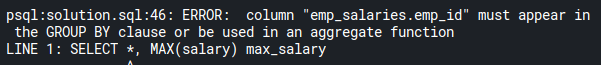This means that the first column of the ` emp_salaries ` table — `emp_id ` column must appear in the ` GROUP BY ` clause or be used in an aggregate function.

To avoid this error, you have two options:

1. A long route using a CTE along with a ` JOIN ` statement.
2. A shorter route using a window function.

The shorter (and preferred) option for us is to take the window function route and write the following query:

``````SELECT *, MAX(salary) OVER() max_salary
FROM emp_salaries;
``````

With just ` OVER() ` , you can use ` MAX() ` as a window function to aggregate the maximum salary to each record in the table: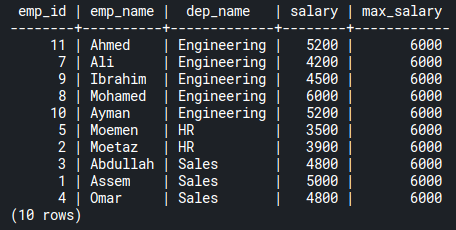### GROUP BYvs.PARTITION BYclauses

What if you want a breakdown of max salaries across each department?

``````SELECT MAX(salary) max_salary
FROM emp_salaries e
GROUP BY dep_name;
``````

This query gets only the maximum salary across each department: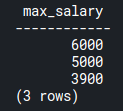Here, there is one column that represents the max salary per department. Again you wouldn’t easily be able to combine the other columns with the max_salary column unless you use window functions.

To compare each record with its corresponding maximum salary by department, use ` PARTITION BY ` :

``````SELECT *,
MAX(salary) OVER(PARTITION BY dep_name) max_salary
FROM emp_salaries;
``````

This query results in the following records:As you can see, the aggregate is done over the department names. So you get a breakdown of the max salary of each department next to each record in the ` emp_salaries ` table.

If you’re still confused about the difference between ` GROUP BY ` and ` PARTITION BY ` , remember this: ` GROUP BY ` reduces the number of rows returned by rolling them up to calculate the aggregate function for each row. `PARTITION BY ` partitions the window based on a specific column; thus it doesn’t affect the number of rows in the result.

### MAX(),MIN(),AVG(),SUM(),COUNT()functions

It’s important to note that the previous examples that you used with `MAX() ` can also be applied with either ` MIN() ` , ` SUM() ` , or ` COUNT() ` . These are other aggregate functions and can also be used as window functions as follows:

``````SELECT
emp_id,
salary,
MAX(salary) OVER(PARTITION BY dep_name) max_salary,
MIN(salary) OVER(PARTITION BY dep_name) min_salary,
SUM(salary) OVER(PARTITION BY dep_name) total_salaries,
COUNT(*) OVER(PARTITION BY dep_name) emp_count
FROM emp_salaries;
``````

which outputs the following result: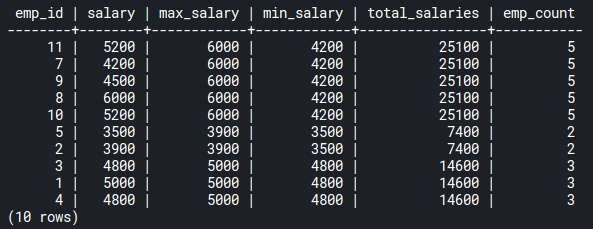While we don’t cover ` AVG() ` here, you can find more information on it in the PostgreSQL docs here .

### ROW_NUMBER()function

The ` ROW_NUMBER() ` is another useful window function. It assigns serial numbers to records according to the window. See the following example:

``````SELECT *,
MAX(salary) OVER(
PARTITION BY dep_name
) max_salary,
ROW_NUMBER() OVER() row_num
FROM emp_salaries;
``````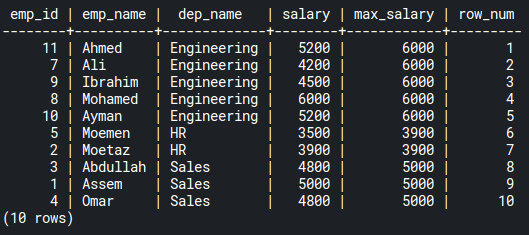As you can see, the row_num column contains an ordered list of numbers across the whole table. That’s because the window here has boundaries across the table, as the ` OVER() ` clause doesn’t have arguments.

A practical use case for ` ROW_NUMBER() ` is to get the most senior employee in each department. Try it on your own and see what you get.

``````SELECT
*,
MAX(salary) OVER(PARTITION BY dep_name) max_salary,
ROW_NUMBER() OVER(PARTITION BY dep_name) seniority
FROM emp_salaries;
``````

Notice that in this case we partition by the department name. That partition would give you a result like this: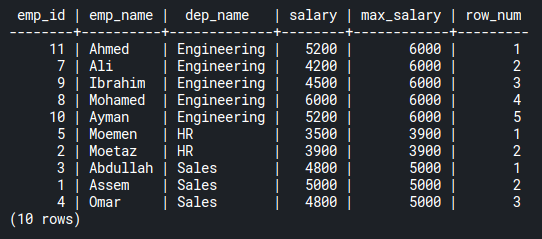As you can see, there is an ordered list according to each department. The engineering department has numbers ` 1 ` to ` 5 ` . HR: ` 1 ` and ` 2 ` . Sales: ` 1 ` to ` 3 ` .

### PARTITION BYandORDER BYclauses

A window function can have ` ORDER BY ` inside an ` OVER() ` clause. To see what that looks like, assume that ` emp_id ` represents seniority and that an ` emp_id ` of ` 8 ` precedes ` 9 ` , meaning that the former joined the company before the latter. We will then sort according to the ` emp_id ` to see the first employees who joined each department:

``````SELECT *,
MAX(salary) OVER(
PARTITION BY dep_name
) max_salary,
ROW_NUMBER() OVER(
PARTITION BY dep_name
ORDER BY emp_id
) seniority
FROM emp_salaries;
``````

The seniority column represents ordered numbers according to the `emp_id ` . If you filter by seniority with ` 1 ` values, you get the first employee for each department who joined the company.

Give it a try here using a subquery:

``````SELECT *
FROM (
SELECT *,
MAX(salary) OVER(
PARTITION BY dep_name
) max_salary,
ROW_NUMBER() OVER(
PARTITION BY dep_name
ORDER BY emp_id
) seniority
FROM emp_salaries
) e
WHERE e.seniority < 2;
``````

### RANK()function

The ` RANK() ` window function returns the rank of the current row with gaps. But what do we mean by gaps?

``````SELECT *,
RANK() OVER(
PARTITION BY dep_name
ORDER BY salary DESC
) rank
FROM emp_salaries;
``````

This query returns the ranks of every employee, in descending order, according to each department. Gaps exist when there are duplicate values: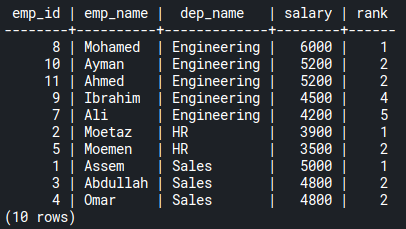As you can see, ` Ayman ` and ` Ahmed ` share the same rank ( ` 2` ) on engineering department salaries. Rank ` 3 ` is a gap, and the ` RANK() ` function skips it. The next rank is ` 4 ` taken by `Ibrahim ` .

### DENSE_RANK()function

` DENSE_RANK() ` window function is similar to ` RANK() ` except that it returns the rank without gaps. If you replace ` RANK() ` with ` DENSE_RANK() ` in the previous query, you’ll find a result query like the following: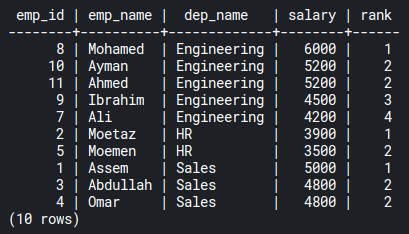Now, rank ` 3 ` is not skipped, as in the case of ` RANK() `.

## Conclusion

Window functions can make you productive. You will spend less time writing your queries with fewer lines of SQL.

In this tutorial, you saw how to use them in PostgreSQL. You learned how to use ` MAX ` , ` MIN ` , ` SUM ` , ` COUNT ` , ` AVG ` , `ROW_NUMBER ` , ` RANK ` , and ` DENSE_RANK ` . There are also other window functions that you can check out in PostgreSQL documentation.